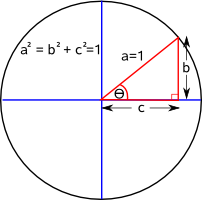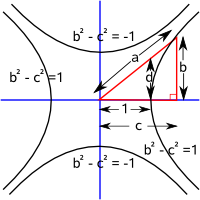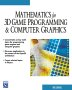# Maths - Trigonometry

Trigonometry is about angles and triangles, there are relationships between the angles and the ratios of the lengths of side of the triangles. These ratios are represented by functions such as sine and cosine, which occur widely in mathematics and physics, even in fields which don't initially appear to be related to physical triangles.

On these pages we will consider two types of trigonometry:

• trigonometry based on a circle where: a²=b²+c²
• trigonometry based on a hyperbola where: a²=b²-c²

For most cases we only need to consider traditional trigonometry based on a circle so feel free to ignore the hyperbolic trigonometry column. Hyperbolic trigonometry starts to become useful when we have a space with the Minkowski norm: (x²-y²) the simplest case is the two dimensional space represented by double numbers as explained here.

## Summary of results

This is a summary of the results in the above pages, for the derivation of these formulae see the links above.

### Right angled triangle

circle based trigonometry hyperbolic trigonometry
Here we will use a unit circle, that is a circle where all the points are exactly 1 unit from the origin, which gives: b²+c²=1. Here we will use a hyperbolic function where the minimum distance from the origin is 1 unit which gives: b²-c²=±1.sine function
sin(θ) = b

hyperbolic sine function
sinh(θ) = b

cosine function
cos(θ) = c
hyperbolic cosine function
cosh(θ) = c
tangent function
tan(θ) = b / c
hyperbolic tangent function
tanh(θ) = d = b/c
cosecant
cosec(θ) = a / b

secant
sec(θ) = a / c

cotangent
cot(θ) = c / b

### Trig Identities

The values of sin, cos and tan are related to each other, for instance,

circle based trigonometry hyperbolic trigonometry
tan θ = sin θ / cos θ tanh θ = sinh θ / cosh θ
cos2θ + sin2θ = 1 cosh2θ - sinh2θ = 1
cosec θ = 1 / sin θ cosech θ = 1 / sinh θ
sec θ = 1 / cos θ sech θ = 1 / cosh θ
cot θ = 1 / tan θ coth θ = 1 / tanh θ

### hyperbolic

sinh(t) = (et - e-t )/2 = (et - 1)/2*et = -i sin(i t)
cosh(t) = (et + e-t )/2 = (et + 1)/2*et = cos(i t)
tanh(t) = (et - e-t )/(et + e-t ) = (e2t - 1)/(e2t + 1)= -i tan(i t)
asinh(x) = ln(x+√(x²+1))
acosh(x) = if (abs(x)<1) ln(x+√(1 - x²))
else ln(x+√(x²-1))
atanh(x) = if (abs(x)<1) 0.5*ln((x-1)/(x+1))
else 0.5*ln((x-1)/(x+1))

### Symmetric and Anti-symmetric Properties

 anti-symmetric sin(θ)= -sin(-θ) sinh(θ)= -sinh(-θ) sin(iθ)= i sinh(θ) symmetric cos(θ)= cos(-θ) cosh(θ)= cosh(-θ) cos(iθ)= cosh(θ) anti-symmetric tan(θ)= -tan(-θ) tanh(θ)= -tanh(-θ) tan(iθ)= i tanh(θ)

### Complimentary and Supplementary Angles

• sin(θ - 90°) = cos(θ)
• cos(θ - 90°) = sin(θ)
• tan(θ - 90°) = cot(θ)

### Non-Right Angled Triangles - The Sine Rule

a / sin A = b / sin B = c / sin C

### Non-Right Angled Triangles - The Cosine Rule

• cos A = (b2 + c2 - a2)/2*b*c
• cos B = (c2 + a 2 - b 2)/2*c*a
• cos C = (a2 + b 2 - c 2)/2*a*b

### Area of triangles

In euclidean space the area of a triangle depends on length of 2 sides and one angle:

area = 1/2 b * c*sin A

This is half the product of two sides and the sin of the included angle.

In hyperbolic space the area depends only on the internal angles:

π-(α+β+γ)=CΔ

circle based trigonometry hyperbolic trigonometry
sin(A+B) = sin A cos B + cos A sin B sinh(A+B) = sinh A cosh B + cosh A sinh B
cos(A+B) = cos A cos B - sin A sin B cosh(A+B) = cosh A cosh B + sinh A sinh B
tan(A+B) = (tan A + tan B)/
(1 - tan A tan B)
tanh(A+B) = (tanh A + tanh B)/
(1 + tanh A tanh B)
sin(A-B) = sin A cos B - cos A sin B sinh(A-B) = sinh A cosh B - cosh A sinh B
cos(A-B) = cos A cos B + sin A sin B cosh(A-B) = cosh A cosh B - sinh A sinh B
tan(A-B) = (tan A - tan B)/
(1 + tan A tan B)
tanh(A-B) = (tanh A - tanh B)/
(1 - tanh A tanh B)

### Products of Trigonometric Functions

circle based trigonometry hyperbolic trigonometry
sin A sin B = 0.5(cos(A-B)-cos(A+B)) sinh A sinh B = 0.5(cosh(A+B)-cosh(A-B))
cos A cos B = 0.5(cos(A-B)+cos(A+B)) cosh A cosh B = 0.5(cosh(A+B)+cosh(A-B))
sin A cos B = 0.5(sin(A-B)+sin(A+B)) sinh A cosh B = 0.5(sinh(A+B)+sinh(A-B))

### Double Angle and Half Angle Formulae

We can derive the double angle from products above where A=B. From this we can then derive the half angle formulae.

circle based trigonometry hyperbolic trigonometry
sin(2A) = 2 sin A cos A sinh(2A) = 2 sinh A cosh A
cos(2A) = cos2A - sin2A = 1 - 2*sin2A cosh(2A) = cosh2A + sinh2A
tan(2A) = 2 tan A/(1 - tan2A) tanh(2A) = 2 tanh A/(1 + tanh2A)
sin(t/2) =±√(0.5 (1- cos(t))) sinh(t/2) =±√(0.5 (cosh(t)-1))
cos(t/2) =±√(0.5 (1+ cos(t))) cosh(t/2) =±√(0.5 (1+ cosh(t)))
tan(t/2) = sin(t)/(1+cos(t)) tanh(t/2) = sinh(t)/(1+cosh(t))

### Angles of Any Magnitude

• sin(0) = 0
• cos(0) = 1
• cos(90° + θ) = -sin(θ)
• cos(90° - θ) = sin(θ)
• sin(180° - θ) = sin(θ)
• sin(180° + θ) = -sin(θ)
• sec(180° + θ) = -sec(θ)
• cosec(180° - θ) = cosec(θ)
• cos(270° + θ) = sin(θ)
• cos(270° - θ) =
• tan(270° + θ) = -cotan(θ)
• tan(270° - θ) = cotan(θ)
• cot(360° + θ) = cot(θ)
• sin(360° - θ) = -sin(θ)

### Series

• sin(a) = a - ( a 3 / 3!) + ( a 5 / 5!) - ( a 7 / 7!) + ...
• cos(a) = 1 - ( a 2 / 2!) + ( a 4 / 4!) - ( a 6 / 6!) + ...
• tan(a) = a + ( a 3 / 3) + ( 2a5 / 15) + ...
• sin-1(x) = x + ( x3 / 3!) + ( 9x5 / 5!) + (225 x7 / 7!) + ...
• cos-1(x) = PI/2 - x - ( x3 / 3!) - (9 x5 / 5!) - ( 225 x7 / 7!) + ...
• tan-1(x) = x - ( x3 / 3) + ( x5 / 5) - ( x7 / 7) + ...

Other topics are as follows:

 triangles relationships between sides and angles in triangles trig functions representing these relationships as continuous functions pythagoras relationship between length of sides of a right angle triangle inverse trig functions finding angle from ratio of sides arc sine, arc cosine, arc tan, etc. rectangular to polar conversion, need for atan2(y,x) function derived functions relationship between trig functions double angle formula series How to calculate the value of trig functions using an infinite series

 metadata block see also: Correspondence about this page Book Shop - Further reading. Where I can, I have put links to Amazon for books that are relevant to the subject, click on the appropriate country flag to get more details of the book or to buy it from them.Mathematics for 3D game Programming - Includes introduction to Vectors, Matrices, Transforms and Trigonometry. (But no euler angles or quaternions). Also includes ray tracing and some linear & rotational physics also collision detection (but not collision response). Other Math Books